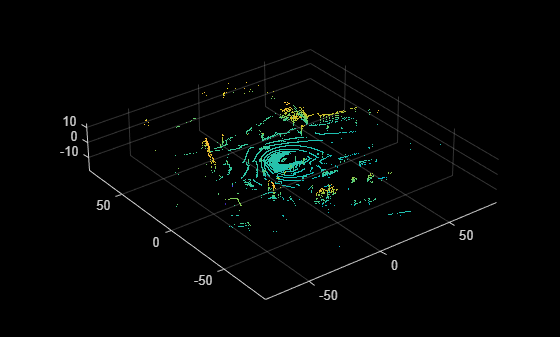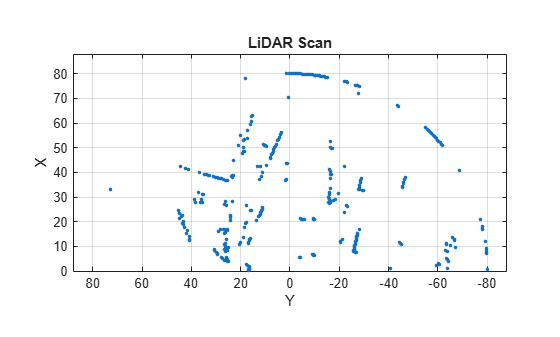# pc2scan

Convert 3-D point cloud into 2-D lidar scan

## Syntax

``scan = pc2scan(ptCloudIn)``
``scan = pc2scan(ptCloudIn,tform)``
``scan = pc2scan(ptCloudIn,Name=Value)``

## Description

example

````scan = pc2scan(ptCloudIn)` converts an input point cloud into a 2-D lidar scan and returns it as a `lidarScan` object.```
````scan = pc2scan(ptCloudIn,tform)` specifies the transformation `tform` of the input point cloud into the 2-D lidar sensor coordinate system, and then converts it into a 2-D lidar scan. `tform` represents the pose of the 2-D lidar sensor with respect to the point cloud origin.```
````scan = pc2scan(ptCloudIn,Name=Value)` specifies options using one or more name-value arguments. For example, `pc2scan(ptCloudIn,ElevationAngleTolerance=5)` selects points with elevation angles in the range [`-5`, `5`] degrees to generate the scan.```

## Examples

collapse all

Create a velodyne PCAP file reader object.

`veloReader = velodyneFileReader("lidarData_ConstructionRoad.pcap","HDL32E");`

Read point cloud data from 0.3 seconds after the start time of the file by using the `readFrame` method. Display the point cloud.

```veloReader.CurrentTime = veloReader.StartTime + seconds(0.3); ptCloud = readFrame(veloReader); pcshow(ptCloud)```Segment the point cloud and remove ground points.

```groundPtsIdx = segmentGroundFromLidarData(ptCloud); nonGroundPtCloud= select(ptCloud,~groundPtsIdx,OutputSize="full");```

Convert the point cloud into 2-D lidar scan and display the output.

```scan = pc2scan(nonGroundPtCloud); figure plot(scan)```## Input Arguments

collapse all

Input point cloud, specified as a `pointCloud` object.

By default, the function assumes the input point cloud is in the sensor coordinate system with the point cloud origin at the sensor center, and the sensor capturing the point cloud has no rotations about the coordinate axes.

You must specify the `Location` property of the `pointCloud` object in meters.

Rigid transformation between the 2-D lidar sensor and the point cloud origin, specified as a `rigidtform3d` object. The function uses `tform` to convert the input point cloud into the 2-D lidar sensor coordinate system.

Note

By default, the function assumes the input point cloud is in the sensor coordinate system, and the sensor capturing the point cloud has no rotations about the coordinate axes if you do not specify `tform`.

### Name-Value Arguments

Specify optional pairs of arguments as `Name1=Value1,...,NameN=ValueN`, where `Name` is the argument name and `Value` is the corresponding value. Name-value arguments must appear after other arguments, but the order of the pairs does not matter.

Example: `pc2scan(ptCloudIn,ElevationAngleTolerance=5)` selects points with elevation angles in the range [`-5`, `5`] degrees to generate the scan.

Elevation angle tolerance, specified as a positive scalar. When generating the 2-D lidar scan, the function selects only the points of the input point cloud with elevation angles in the range [`-ElevationAngleTolerance`, `ElevationAngleTolerance`]. Lower values of `ElevationAngleTolerance` can result in a more accurate output scan. Units are in degrees.

Data Types: `single` | `double` | `int8` | `int16` | `int32` | `int64` | `uint8` | `uint16` | `uint32` | `uint64`

Angle between the consecutive scan lines of the 2-D lidar sensor, specified as a positive scalar. Lower values can result in a finer scan output. Units are in degrees.

Data Types: `single` | `double` | `int8` | `int16` | `int32` | `int64` | `uint8` | `uint16` | `uint32` | `uint64`

Scanning range of the 2-D lidar sensor, specified as a two-element vector of the form `[min max]`. Units are in meters.

Data Types: `single` | `double` | `int8` | `int16` | `int32` | `int64` | `uint8` | `uint16` | `uint32` | `uint64`

Scanning angle limits of the 2-D lidar sensor, specified as a two-element vector of the form `[min max]`. This vector defines the horizontal field of view of the sensor. Units are in degrees.

Data Types: `single` | `double` | `int8` | `int16` | `int32` | `int64` | `uint8` | `uint16` | `uint32` | `uint64`

## Output Arguments

collapse all

Output 2-D lidar scan, returned as a `lidarScan` object.

## Algorithms

The function follows these steps to convert a point cloud into a 2-D lidar scan.

• Converts the input point cloud to the 2-D lidar sensor coordinate system using the specified transformation `tform`. If you do not specify `tform`, the function assumes the data is in the sensor coordinate system.

• Projects the points on the xy-plane. For each projected point, the function computes the angle and range. The angle is counter-clockwise positive along the x-axis, and the range is the distance from the origin.

• Selects the points whose angle and range are within the specified `ScanAngleLimits` and `ScanRangeLimits`, respectively.

• Selects the points whose elevation angle is within the specified `ElevationAngleTolerance`.

• Computes the scan line index for each point from the measured angle. The function assigns a scan angle to each scan line, starting from the first scan line and assigning it the value of the first element of `ScanAngleLimits`. The function then increments the angle in steps of the specified `ScanAngleResolution` to the remaining scan lines.

• By default, each scan line index has the maximum range. For multiple scan lines with same index, the function assigns the range value of the point closest to the origin.

• Generates the 2-D lidar scan using the scan angles and the range values.

## Version History

Introduced in R2022a

expand all